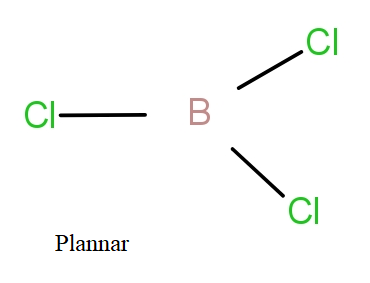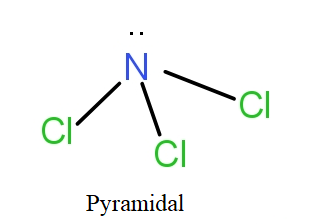$BC{l_3}$ is a planar molecule, while $NC{l_3}$ is pyramidal, because :a.) N—Cl bond is more covalent than B—Cl bond.b.) Nitrogen atom is smaller than boron atomc.) B—Cl bond is more polar than N—Cl bond.d.) $BC{l_3}$ has no lone pair of electrons but $NC{l_3}$ has a lone pair of electrons.Verified
145.8k+ views
Hint: Boron is electron deficient species while nitrogen is not. $BC{l_3}$ has $s{p^2}$ hybridisation with electronic configuration of boron = $1{s^2}2{s^2}2{p^1}$while $NC{l_3}$ has $s{p^3}$ hybridisation with electronic configuration of N = $1{s^2}2{s^2}2{p^3}$.

To get the answer to this, we need to know about planar and pyramidal shapes.
Planar is the geometry when all atoms are in the same plane while pyramidal shape comes under tetrahedral geometry. The tetrahedral geometry involves four ligands present tetrahedrally w.r.t. the central metal atom. They have bond angle of ${109.4^0}$ between adjacent ligands.
If we see $BC{l_3}$ and $NC{l_3}$, then the Boron atom has electronic configuration as-
B = $1{s^2}2{s^2}2{p^1}$
The 2s and 2p orbital will hybridise giving $s{p^2}$ hybrid orbitals that will form bonds with chlorine atoms. The boron with $s{p^2}$ hybridisation has three bond pairs and no lone pair. So, these three bond pairs form planar structure with ${120^0}$ bond angle.
In case of $NC{l_3}$, the nitrogen atom has electronic configuration as-
N = $1{s^2}2{s^2}2{p^3}$
The 2s and 2p orbitals of nitrogen hybridise giving four $s{p^3}$ hybrid orbitals that will form bond pairs with chlorine. The nitrogen with $s{p^3}$ hybridisation has three bond pairs and one lone pair. In actuality, it has tetrahedral geometry with three sides of the tetrahedral having a bond pair and one side there is lone pair. On ignoring the lone pair, visibly the structure is of pyramid shape. Thus, named so.So, the correct answer is “Option D”.

Note: The geometry and shape of a molecule depends upon the number of bond pairs and number of lone pairs present in the molecule. There are bond pair-bond pair repulsions, bond pair-lone pair repulsions and lone pair-lone pair repulsions which may distort the geometry giving new shapes.